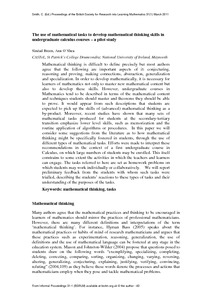# The use of mathematical tasks to develop mathematical thinking skills in undergraduate calculus courses – a pilot study

Breen, Sinead and O'Shea, Ann (2011) The use of mathematical tasks to develop mathematical thinking skills in undergraduate calculus courses – a pilot study. Proceedings of the British Society for Research into Learning Mathematics, 31 (1). pp. 43-48.Previewmore...Add this article to your Mendeley library

## Abstract

Mathematical thinking is difficult to define precisely but most authors agree that the following are important aspects of it: conjecturing, reasoning and proving, making connections, abstraction, generalization and specialization. In order to develop mathematically, it is necessary for learners of mathematics not only to master new mathematical content but also to develop these skills. However, undergraduate courses in Mathematics tend to be described in terms of the mathematical content and techniques students should master and theorems they should be able to prove. It would appear from such descriptions that students are expected to pick up the skills of (advanced) mathematical thinking as a by-product. Moreover, recent studies have shown that many sets of mathematical tasks produced for students at the secondary-tertiary transition emphasize lower level skills, such as memorization and the routine application of algorithms or procedures. In this paper we will consider some suggestions from the literature as to how mathematical thinking might be specifically fostered in students, through the use of different types of mathematical tasks. Efforts were made to interpret these recommendations in the context of a first undergraduate course in Calculus, on which large numbers of students may be enrolled. This itself constrains to some extent the activities in which the teachers and learners can engage. The tasks referred to here are set as homework problems on which students may work individually or collaboratively. We will report preliminary feedback from the students with whom such tasks were trialled, describing the students’ reactions to these types of tasks and their understanding of the purposes of the tasks.

Item Type: Article mathematical thinking; mathematical tasks; undergraduate; calculus courses; Faculty of Science and Engineering > Mathematics and Statistics 4905 Dr. Ann O'Shea 17 Apr 2014 12:28 Proceedings of the British Society for Research into Learning Mathematics BSRLM NoItem control page